# Uniform tilings

Steven Dutch, Professor Emeritus, Natural and Applied Sciences, University of Wisconsin - Green Bay

tilingswith regular polygons are said to be k-uniform if there are precisely k different kinds of vertices in the tiling.

## 1-Uniform (Archimedean) tilings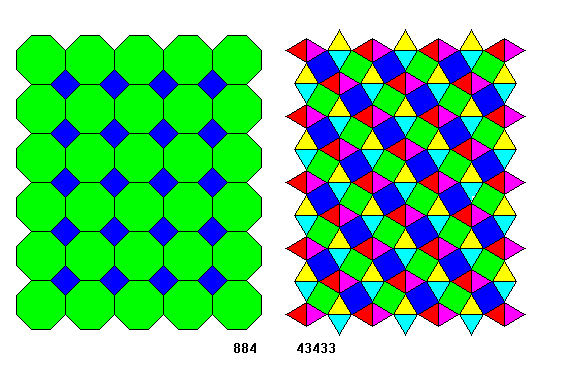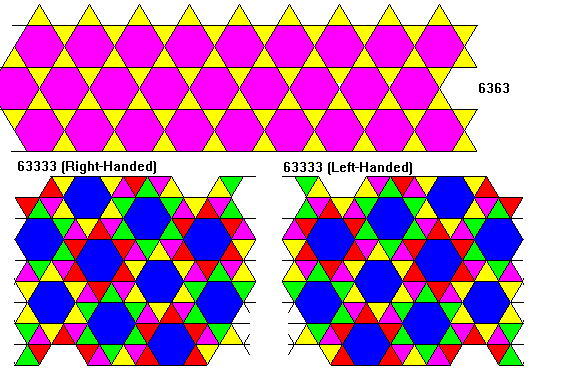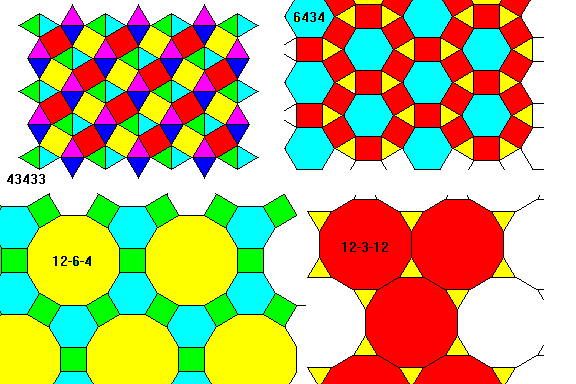## 2-Uniform tilings

There are 20 tilings with regular polygons that are 2-uniform, that is, have two kinds of vertices. Each is shown twice: once to illustrate the different vertices and again as a colored pattern.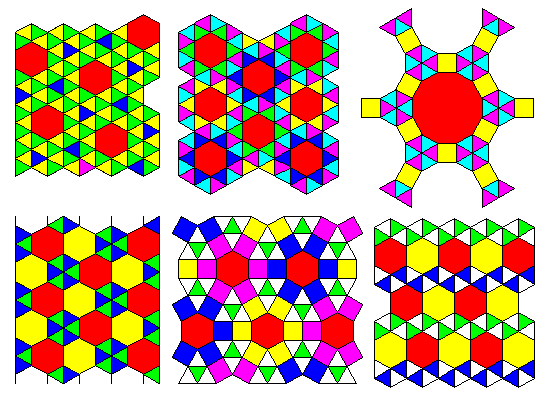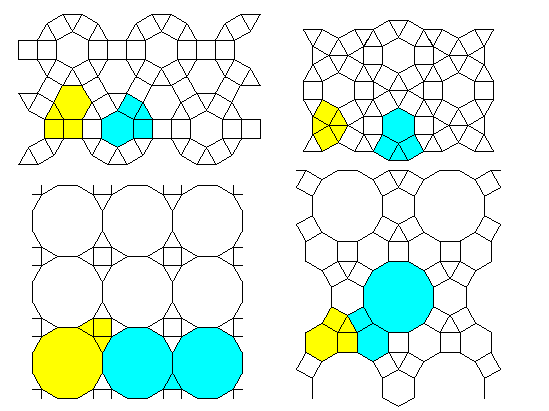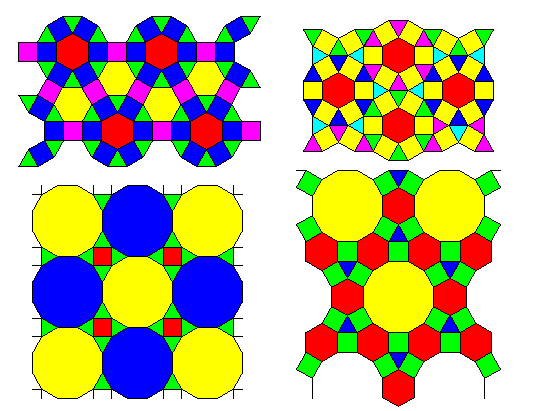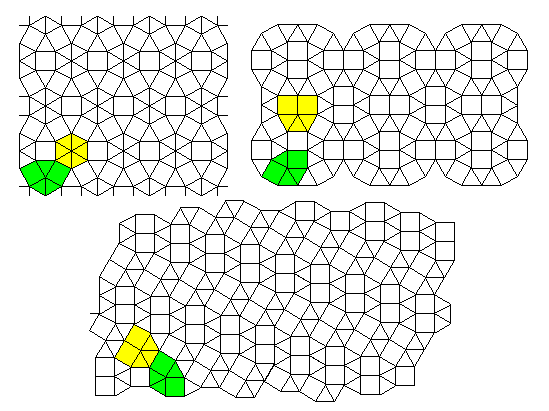## 3 and Higher-Uniform tilings

Once we go beyond k=2 the problem of discovering tilings gets more complex. For example, consider the tiling where a row of triangles alternates with three rows of squares. There are two types of vertex: 33344 and 4444. Now consider the tiling with four rows of squares. We still only have 33344 and 4444 vertices, but some of the 4444's share an edge with a 33344, but others share edges only with other 4444's. Do we have two or three different vertices?

Mathematician Otto Krotenheerdt enumerated the tilings where there are k distinct types of vertices. That rules out the example above where we have two kinds of 4444 vertices. He found that for each k the number of tilings was;

• k=1; 11 tilings
• k=2; 20 tilings
• k=3; 39 tilings
• k=4; 33 tilings
• k=5; 15 tilings
• k=6; 10 tilings
• k=7; 7 tilings
• k=8 and greater; no tilings

For k=1 and 2 his results are the same as the tilings shown. For k = 3 and above his definition is more restrictive. Does it seem surprising that for k=8 and greater he finds no tilings? It actually makes sense. There are only 11 kinds of vertex that can occur in a plane tiling with regular polygons - the Archimedean vertices, so obviously k cannot exceed 11 in any case. Vertex 488 can only occur in the 488 tiling - there is no way to substitute any other polygons, so the number of possible different vertices drops to 10. It shouldn't be terribly surprising that we can't squeeze all ten into a single tiling, or even as many as eight types.

If we allow tilings where vertices can be the same type as long as they are in topologically different settings, there is no limit to k. Even for k=4 a complete enumeration has not been done, nor is there any way of estimating the number of tilings for large k.

## References

Branko Grunbaum and G.C. Shephard, 1989; tilingsand Patterns, an Introduction, Freeman, 446p. sections 2.1 and 2.2

Created 2 July. 1999, Last Update 2 July. 1999